# Correlation plot in R with corPlot

William Revelle

## `corPlot` function from `psych` package

The `corPlot` function is very useful for visualizing a correlation matrix. Consider the `longley` data set and pass some of its columns to the function. You could also use the `select` argument to select the subset of variables.

``````# install.packages("psych")
library(psych)

corPlot(longley[, 2:5])``````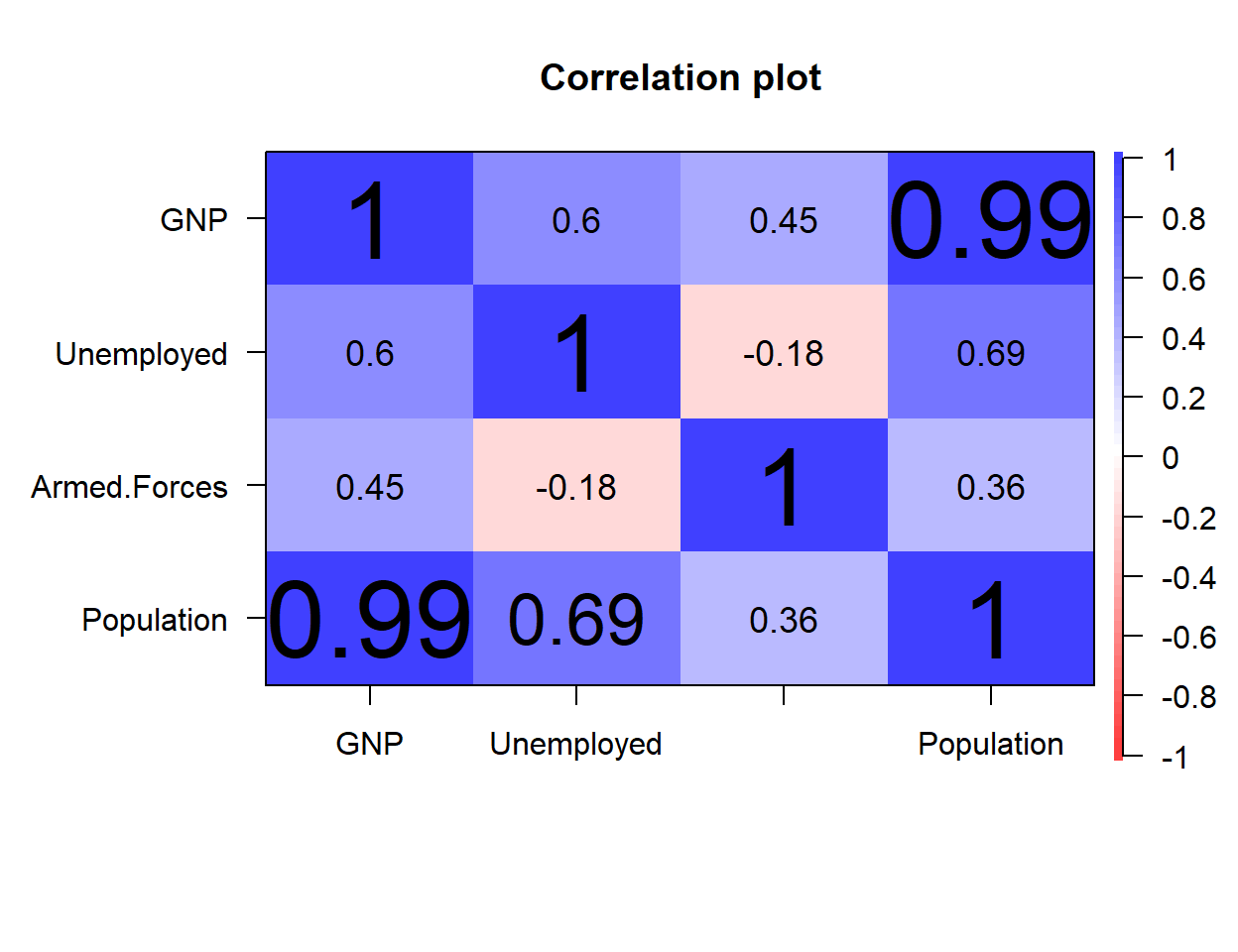By default, the function display the full names of the columns (if fit). If you want to display only some letters set `min.length` to the desired number. You can also set custom labels with `labels`.

``````# install.packages("psych")
library(psych)

corPlot(longley[, 2:5],
min.length = 3)``````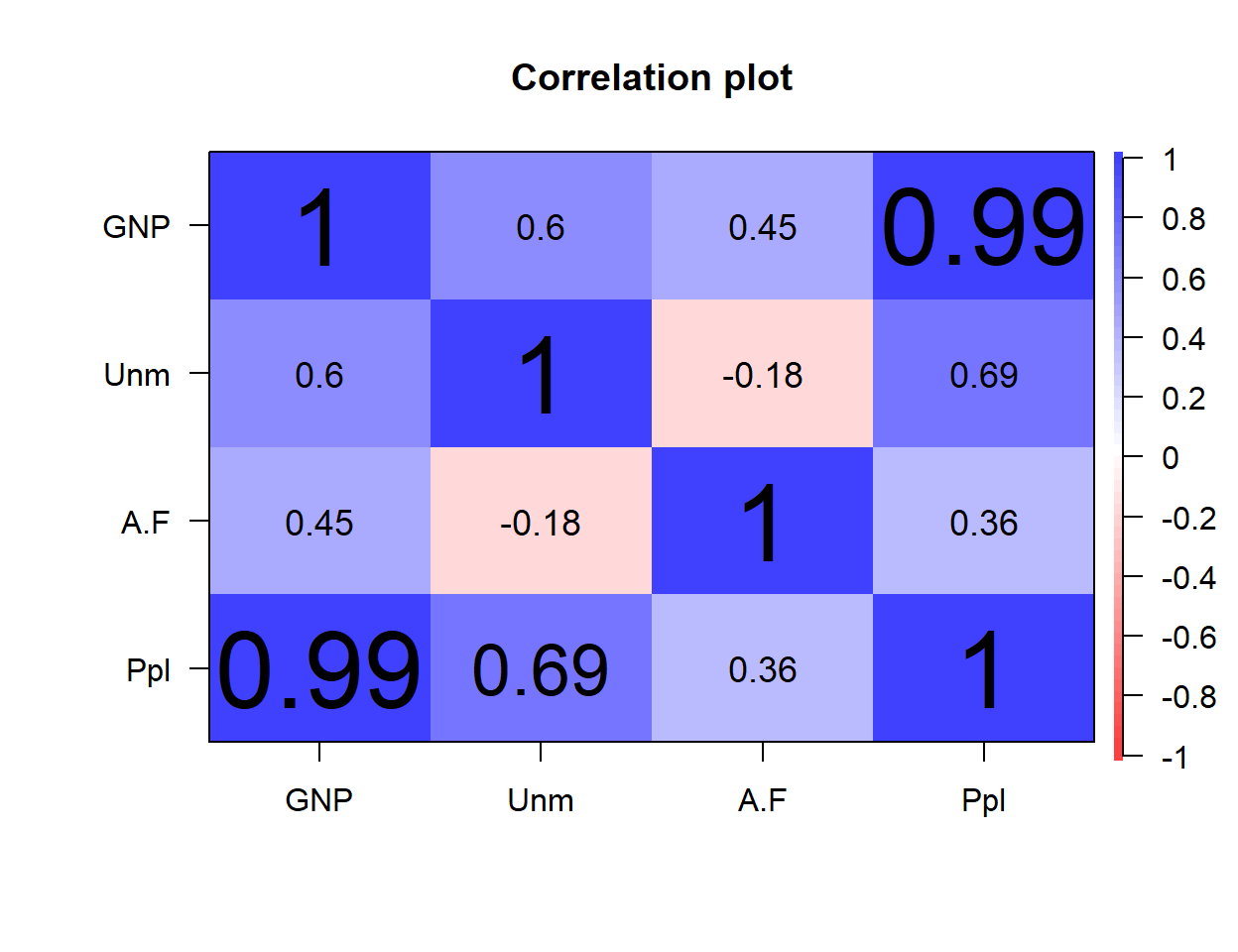### Correlation values and scales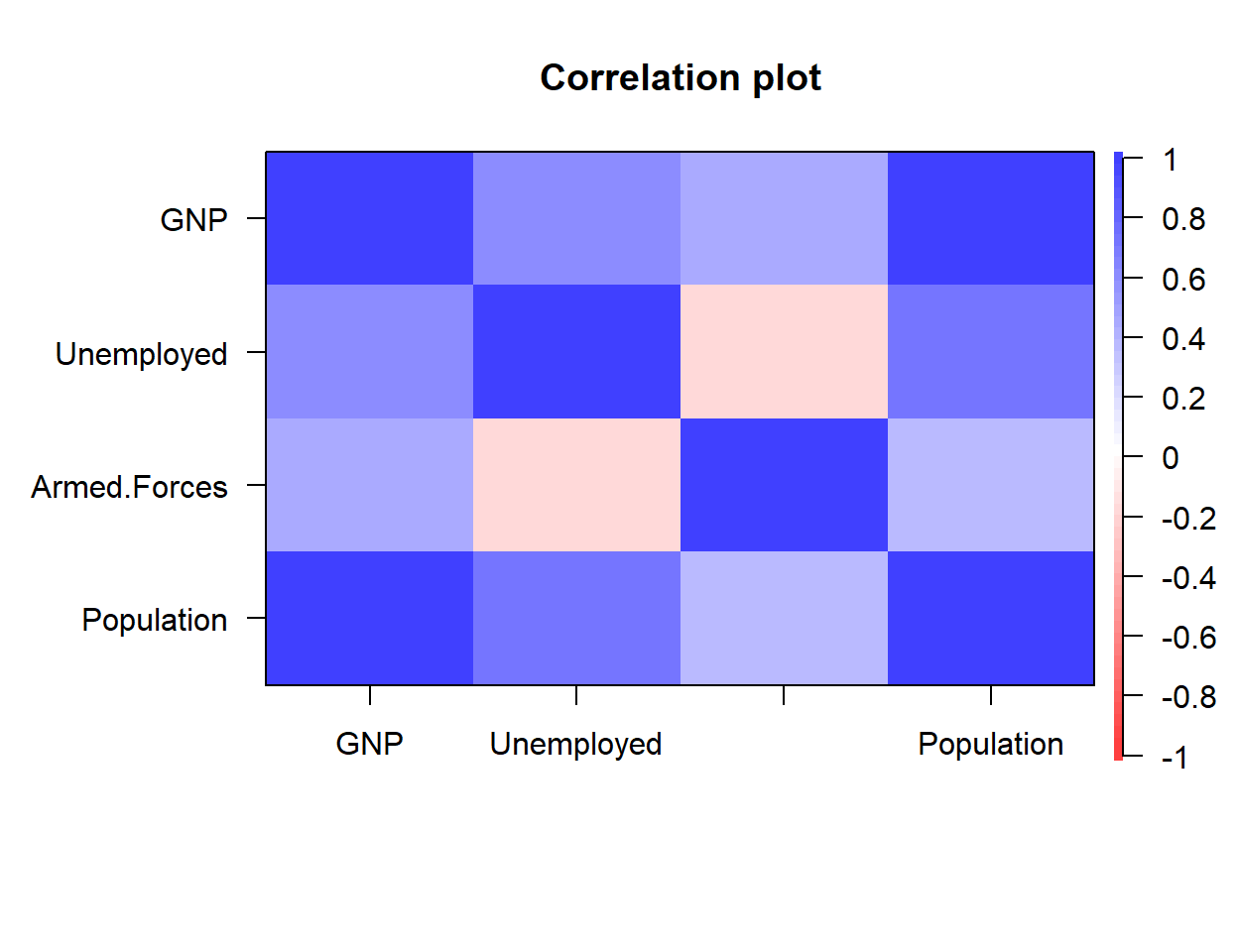No correlation displayed

Set `numbers = FALSE` to avoid displaying the correlation values.

``````# install.packages("psych")
library(psych)

corPlot(longley[, 2:5],
numbers = FALSE)``````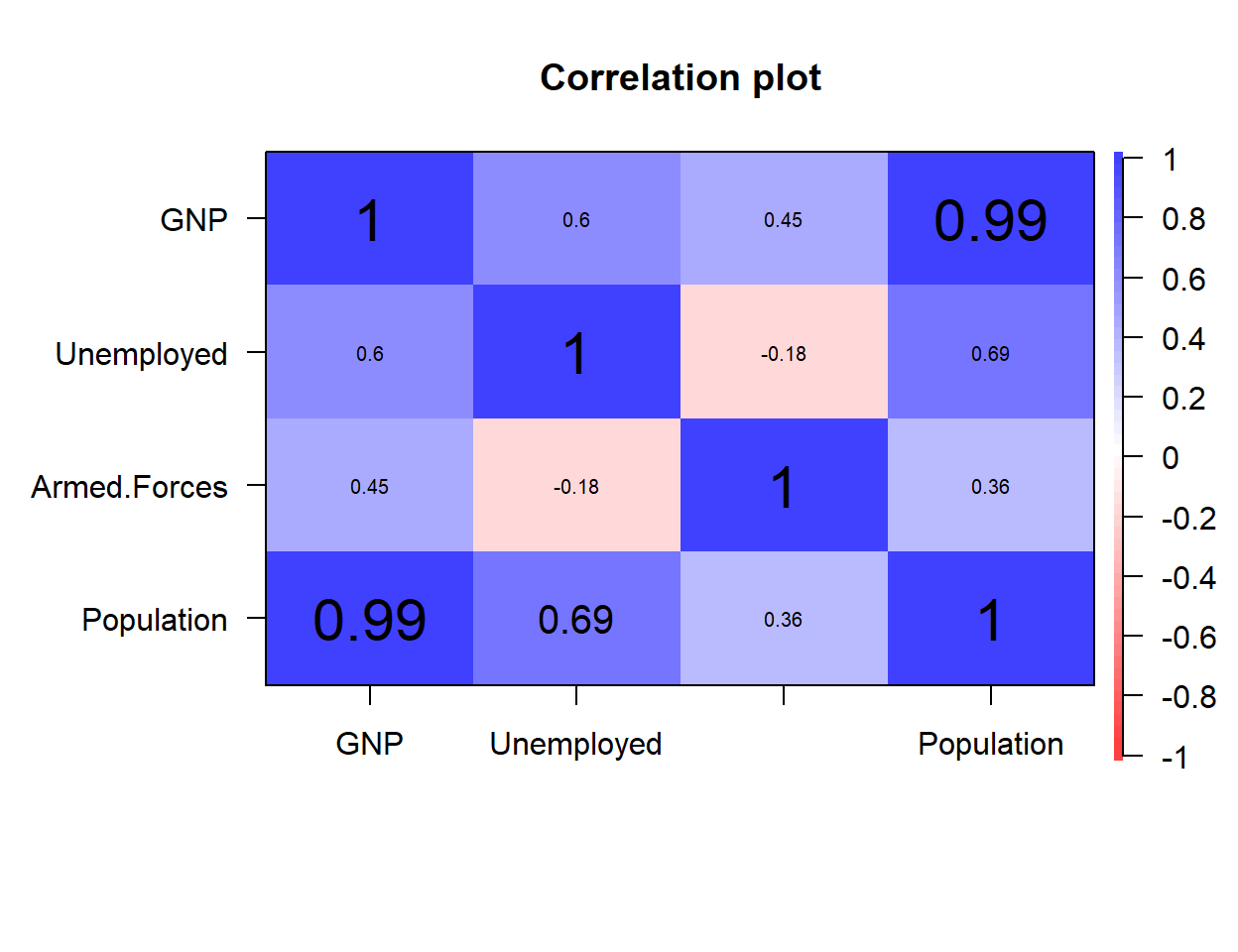Correlation text size

The default text size is too big for some use cases. Modify the font size with `cex`.

``````# install.packages("psych")
library(psych)

corPlot(longley[, 2:5],
cex = 1.25)``````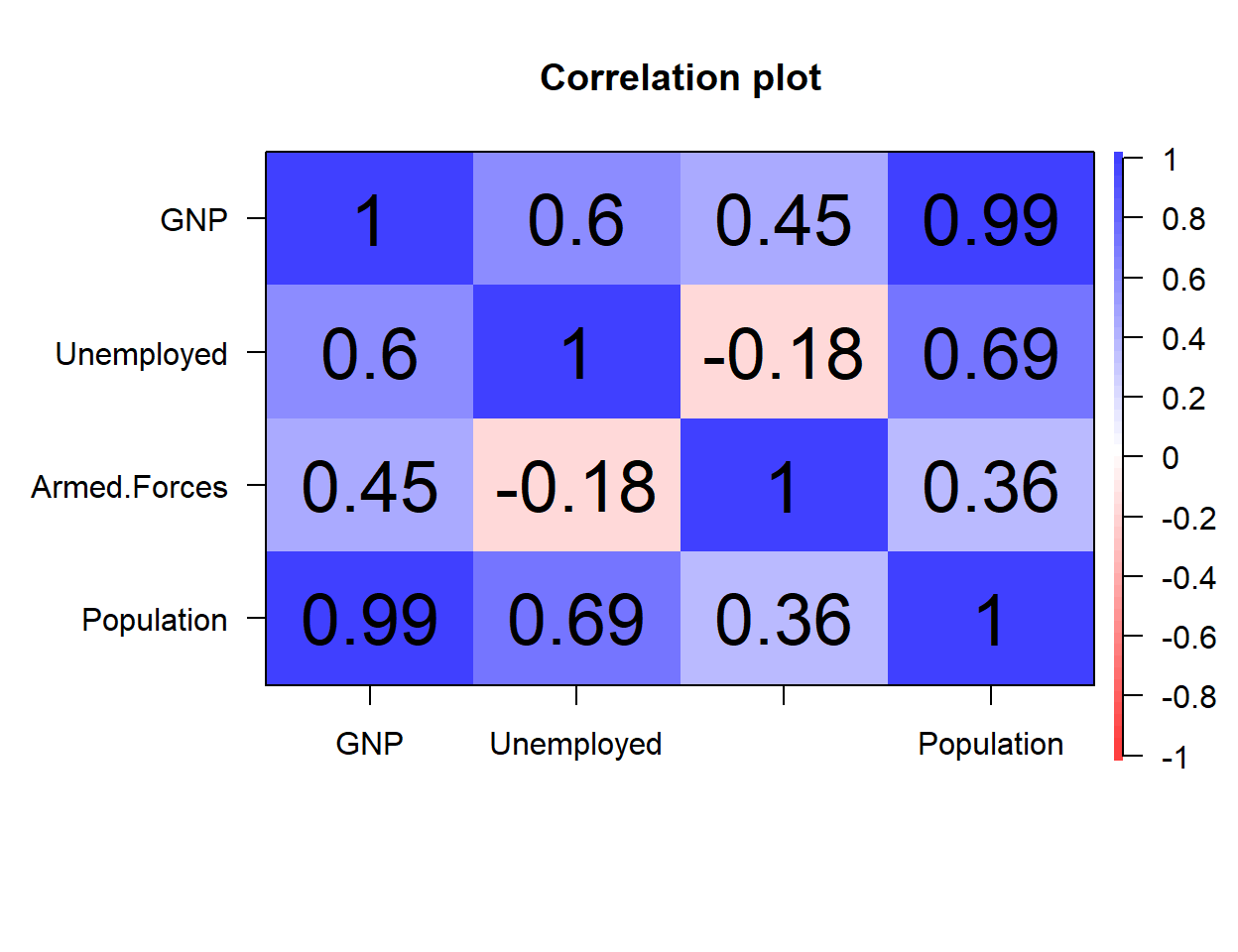Correlation text of the same size

If you don’t like the scaled correlations text set `scale = FALSE`.

``````# install.packages("psych")
library(psych)

corPlot(longley[, 2:5],
scale = FALSE)``````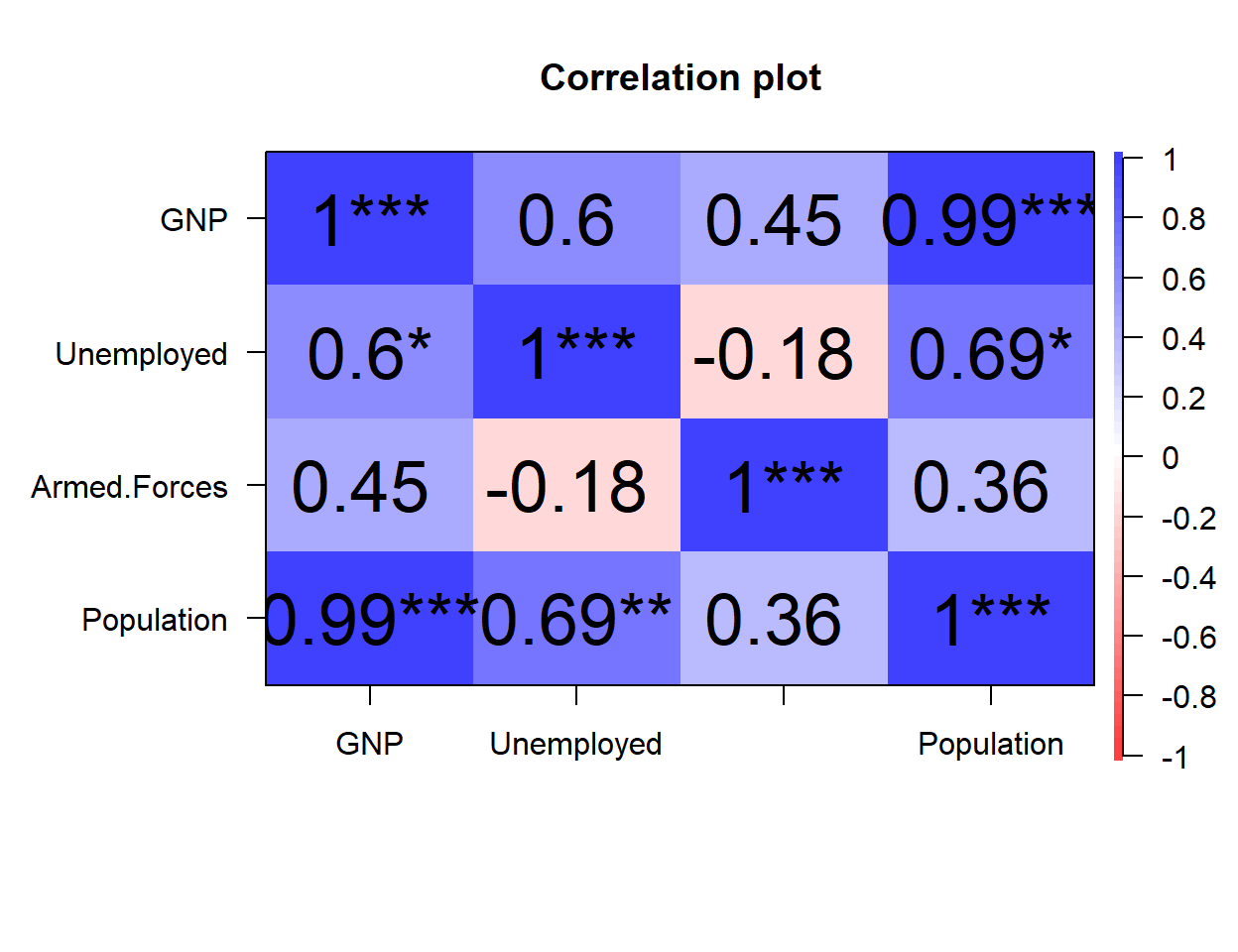Correlation with significance levels

Show significance levels with astricks setting `stars = TRUE`.

``````# install.packages("psych")
library(psych)

corPlot(longley[, 2:5],
stars = TRUE)``````Correlation scaled to p-values

If you prefer scaling the correlation texts to p-values instead of correlation set `pval = TRUE`.

``````# install.packages("psych")
library(psych)

corPlot(longley[, 2:5],
pval = TRUE)``````

### Diagonal and upper panel

Remove the diagonal

It is possible to remove the diagonal of the correlation matrix with `diag = FALSE`.

``````# install.packages("psych")
library(psych)

corPlot(longley[, 2:5],
diag = FALSE)``````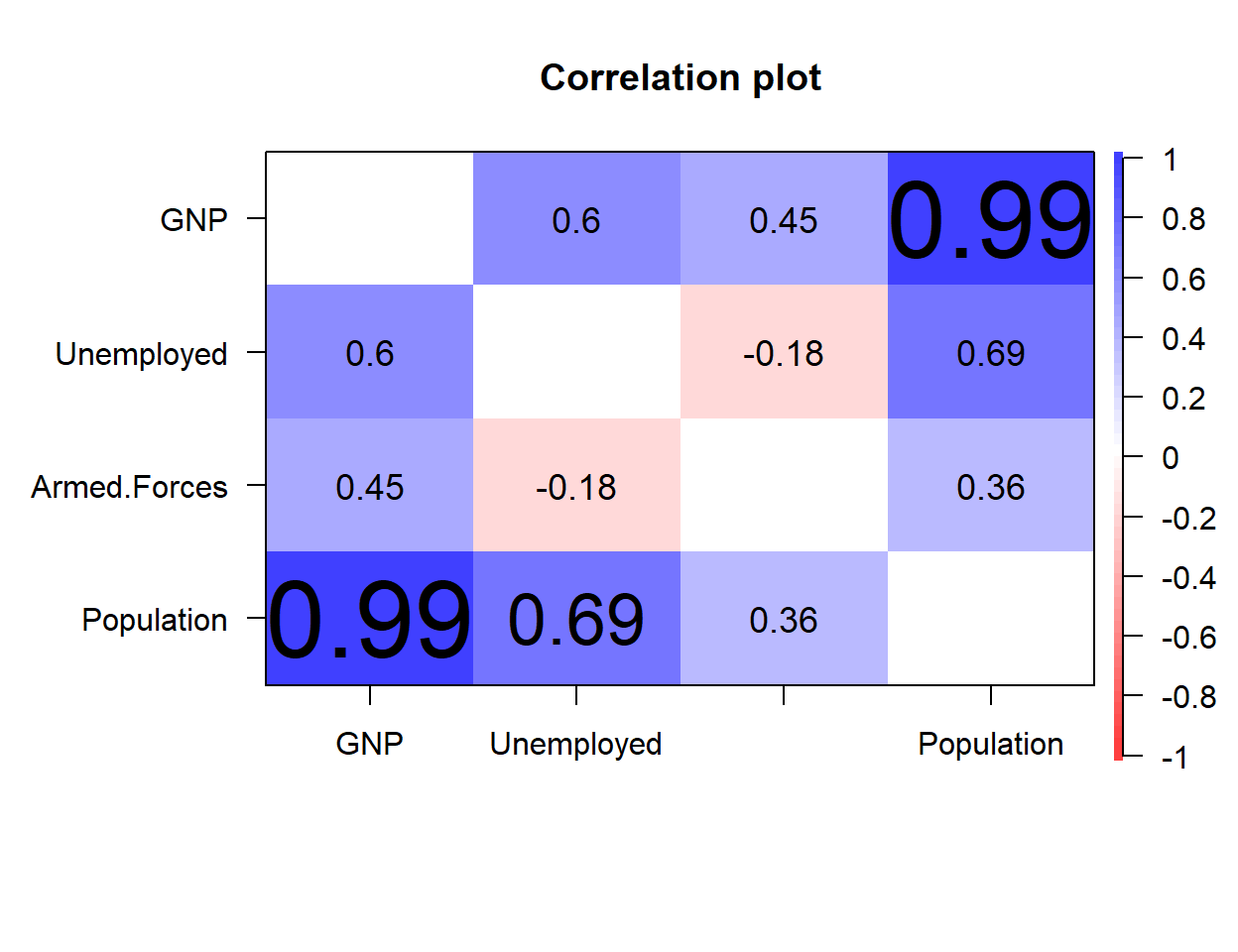Remove the upper panel

You can also remove the upper panel with `upper = FALSE`. Note that the plot box will still displayed.

``````# install.packages("psych")
library(psych)

corPlot(longley[, 2:5],
upper = FALSE)``````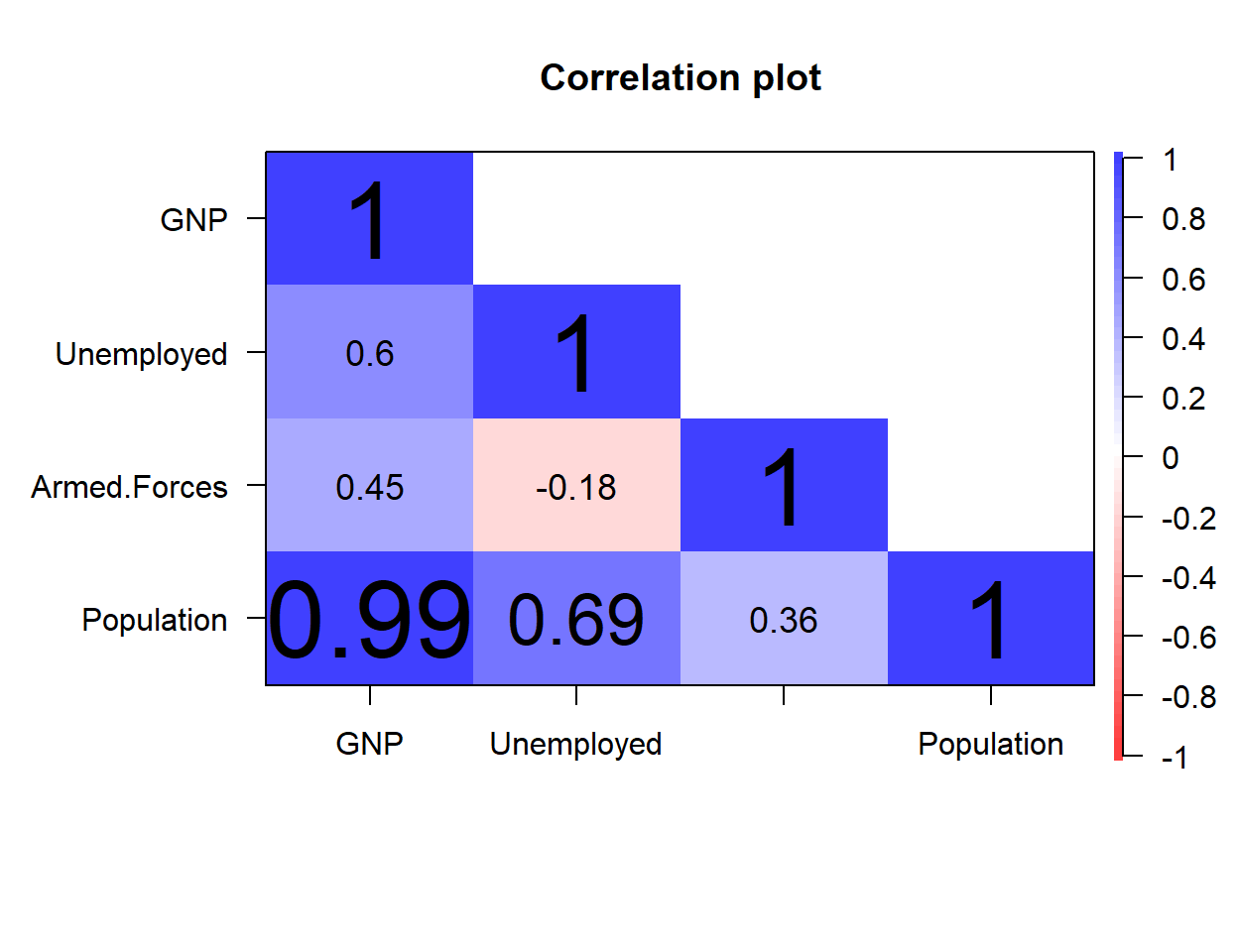### Color palette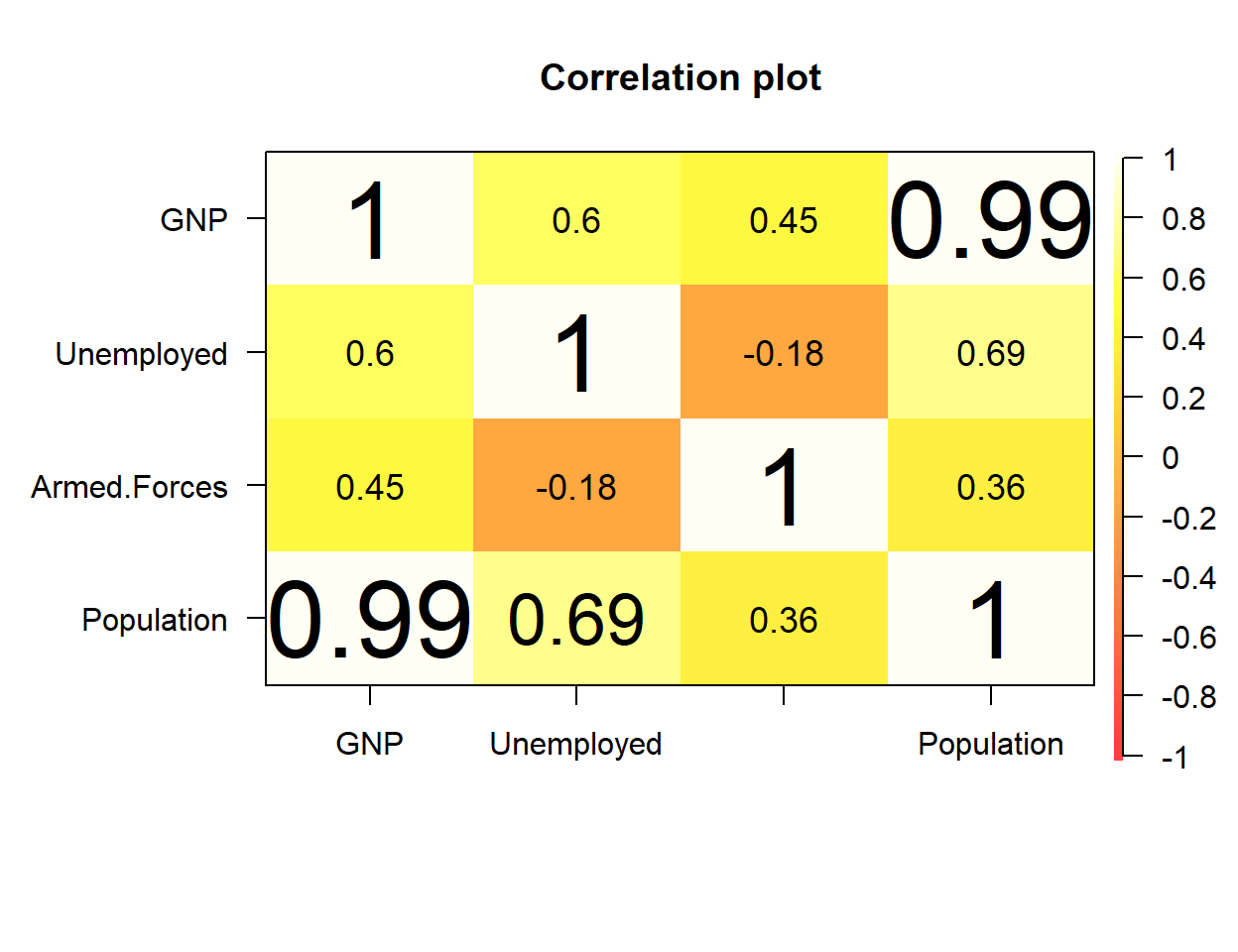The default color palette can be customized passing a color ramp palette to `gr` argument as in the example below.

``````# install.packages("psych")
library(psych)

corPlot(longley[, 2:5],
gr = colorRampPalette(heat.colors(40)))``````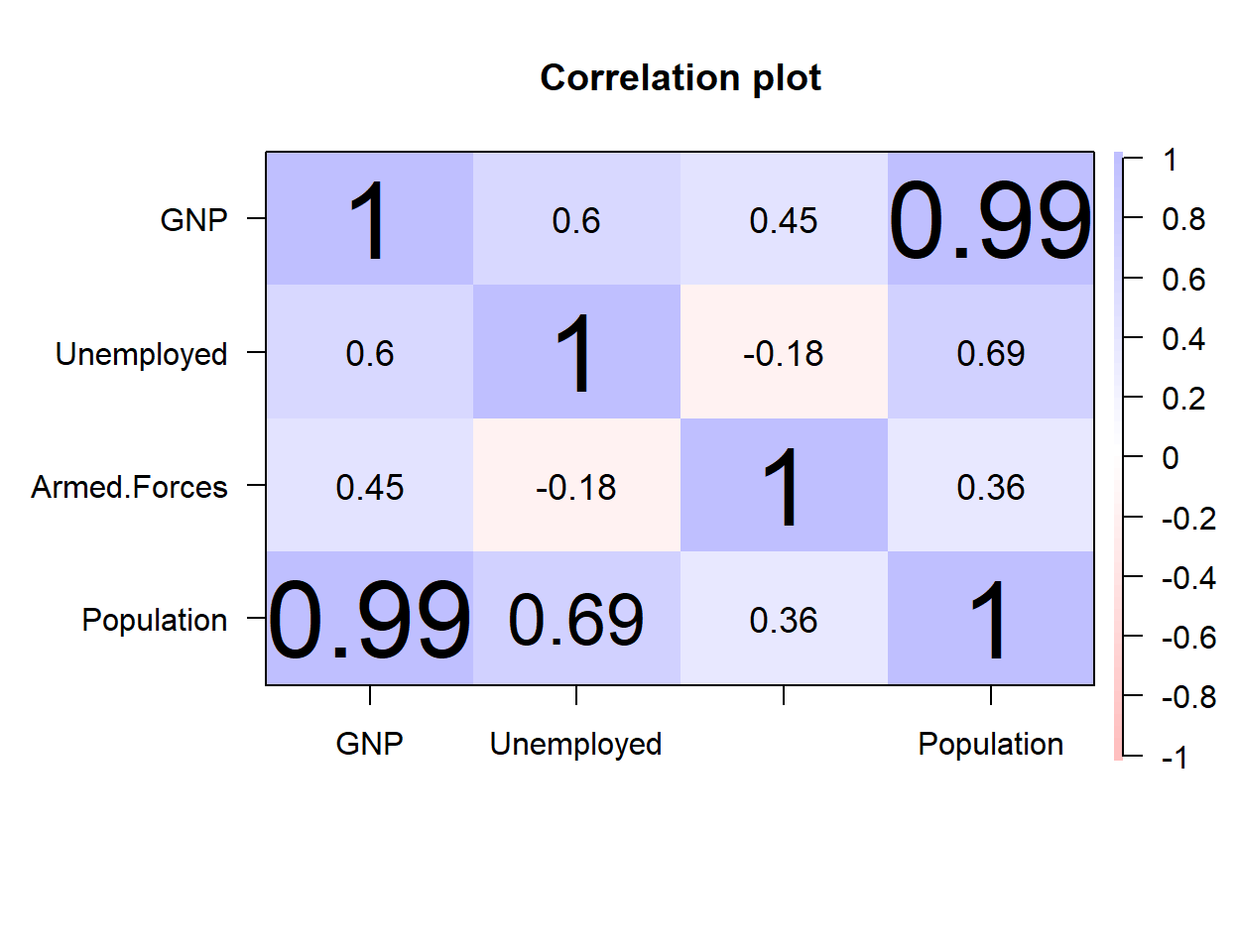Note that the transparency of the color palette can also be customized with `alpha`. The default value is `alpha = 0.75`.

``````# install.packages("psych")
library(psych)

corPlot(longley[, 2:5],
alpha = 0.25)``````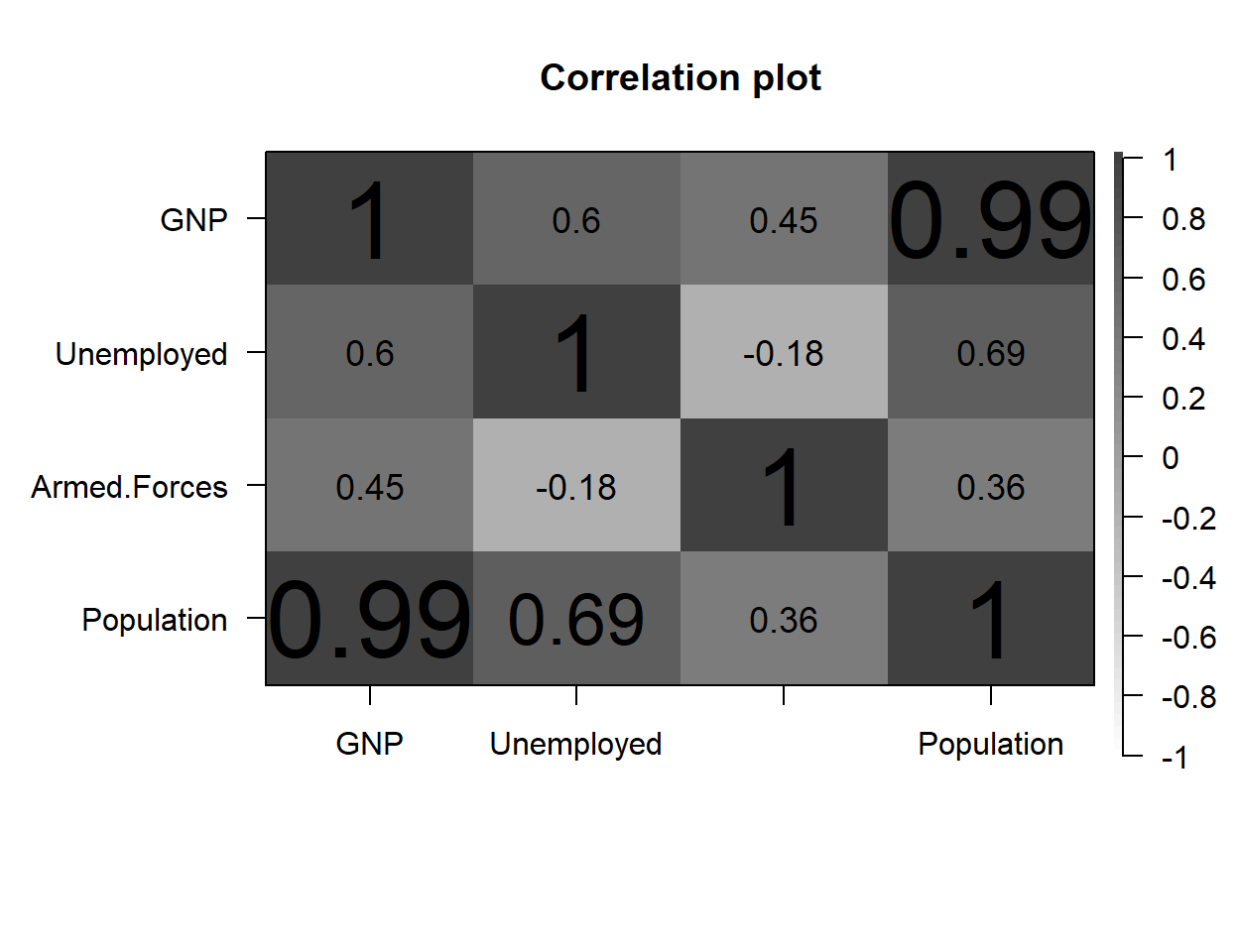There is also an argument named `colors` which converts the default color palette to a gray scale.

``````# install.packages("psych")
library(psych)

corPlot(longley[, 2:5],
colors = FALSE)``````